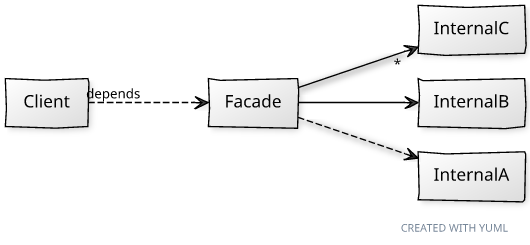## Friday, January 29, 2010

### Practical Php Patterns: Facade

This post is part of the Practical Php Pattern series.

The structural pattern of today is the Facade one.
A Facade is a class that provides a unified interface to a subsystem, totally abstracting away the concrete classes which compose it. The Client is thus decoupled from the internals of the Facade's module.
This pattern is usually implemented to reduce coupling between subsystems and towards vendor code; libraries often provide a Facade to hide their internals and being able to change them in subsequent releases (e.g. the Doctrine static class in Doctrine 1.x is a Facade, and the EntityManager one is its equivalent in Doctrine 2; the Facade remained unchanged in every release of the same branch.)There are some important glitches you have to avoid while implementing a Facade:
• it should not be a Singleton or a static class; otherwise the global state of the subsystem will be hidden under the Facade, effectively preventing isolation of tests that involve. Imagine test against a database schema you cannot ever reset. Doctrine 1 was fundamentally flawed from this point of view.
• The Facade should only return and accept interfaces or value objects in its method signatures, so that there are no transitive dependencies. If the Facade gives away references to the internal collaborators of its module, it won't achieve its decoupling goal.
The job that the code sample accomplishes is outrageously simple. I know some readers would prefer a real world situation, but often the domain details obscurate the intent of the described pattern. That's why programming languages manuals start with Hello World programs and not with real world situations which bring in thousands of lines of code.
```<?php
// Various classes we want to shield the Client from.
class Adder
{
public function add(\$a, \$b)
{
return \$a + \$b;
}
}

class Subtractor
{
public function subtract(\$a, \$b)
{
return \$a - \$b;
}
}

class Multiplier
{
public function multiply(\$a, \$b)
{
return \$a * \$b;
}
}

class Divider
{
public function divide(\$a, \$b)
{
if (\$b == 0) {
throw new Exception('Division by zero.');
}
return \$a / \$b;
}
}

// Client code
\$adder = new Adder();
echo '254 + 113 = ', \$adder->add(254, 113), "\n";
\$divider = new Divider();
echo '256 / 8 = ', \$divider->divide(256, 8), "\n";
```
Application of a Facade class to the previously presented code:
```<?php
include_once 'BeforeFacade.php';

class CalculatorFacade
{
public function __construct(Adder \$adder,
Subtractor \$subtractor,
Multiplier \$multiplier,
Divider \$divider)
{
\$this->_adder = \$adder;
\$this->_subtractor = \$subtractor;
\$this->_multiplier = \$multiplier;
\$this->_divider = \$divider;
}

public function calculate(\$expression)
{
list (\$a, \$operator, \$b) = explode(' ', \$expression);
// eliminating switch constructs is not in the intent of this pattern
switch (\$operator) {
case '+':
return \$this->_adder->add(\$a, \$b);
break;
case '-':
return \$this->_subtractor->subtract(\$a, \$b);
break;
case '*':
return \$this->_multiplier->multiply(\$a, \$b);
break;
case '/':
return \$this->_divider->divide(\$a, \$b);
break;
}
}
}

class CalculatorFactory
{
public function getCalculator()
{
return new CalculatorFacade(new Adder(), new Subtractor(), new Multiplier(), new Divider());
}
}

// Client code
\$calculatorFactory = new CalculatorFactory;
\$calculator = \$calculatorFactory->getCalculator();
echo '254 + 113 = ', \$calculator->calculate('254 + 113'), "\n";
echo '256 / 8 = ', \$calculator->calculate('256 / 8'), "\n";
```
In the example, the library vendor or the component's owner is now free to change the internal concrete classes. Moreover, he can still change signatures and move methods between classes, a feature that even by using Abstract Factories to return Adder and its sibling classes cannot be achieved. Only external interfaces are published, while the contracts between internal classes are not refererred to in any way, stimulating internal refactoring and shifting of responsibilities between classes. For instance, we can easily merge or extract classes and introduce collaborators without the Client code being affected.
Facade is one of the most powerful decoupling patterns because it hides every unnecessary assumption beyond a heavy curtain, thus preventing change in a software module, which will certainly happen, from spreading in the whole application.

#### 5 comments:

Anonymous said...

Great job on this series!

mauro said...

how do you pronounce "façade"?

/fakáde/ or /fasád/ ?

Giorgio said...

Both spellings should be pronounced as /fasad/:
http://en.wiktionary.org/wiki/facade

Unknown said...

I am a newer and I can't understand well for "should only return and accept interfaces or value objects"，could you give a case？

Thank you！

Giorgio said...

Hi, what I mean is: the point of a Facade is to hide the complexity of the object graph behind it, and break dependencies from your code to those classes.
So if in the interface you have to build other objects from that graph, it defeats the point. Only passing in Value Objects (or even primitive types) is a good rule of thumb to avoid that.